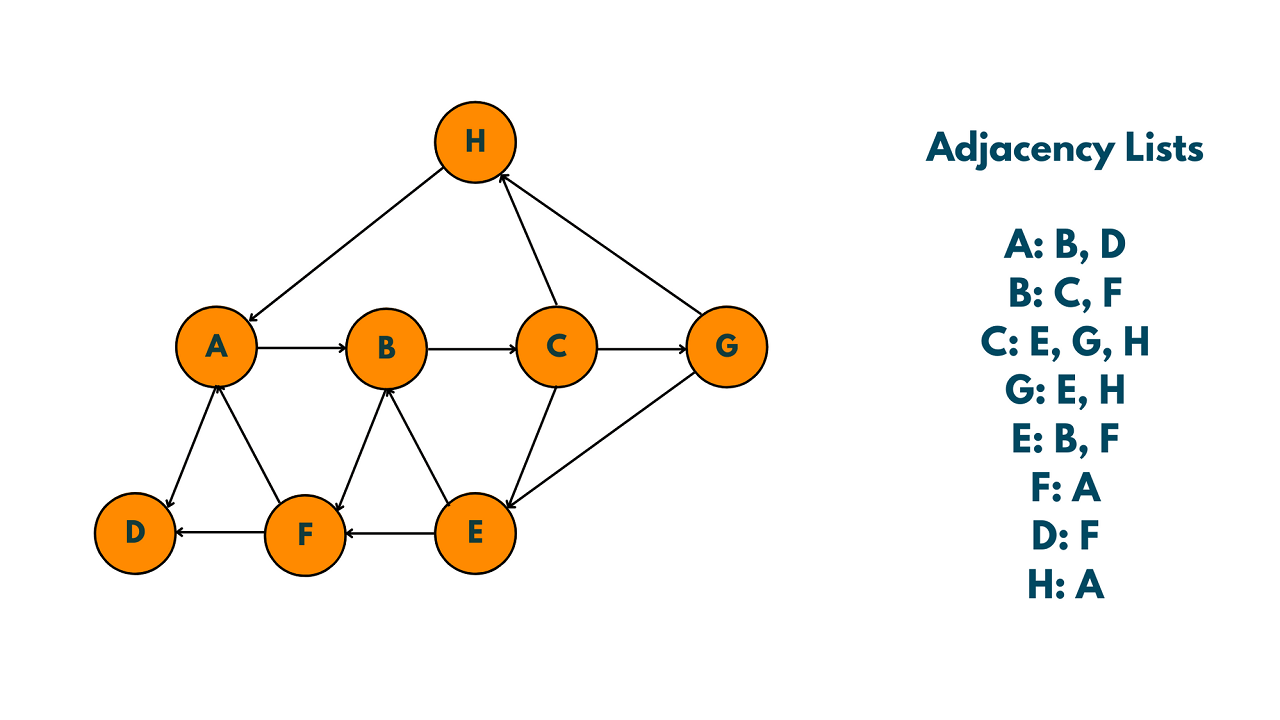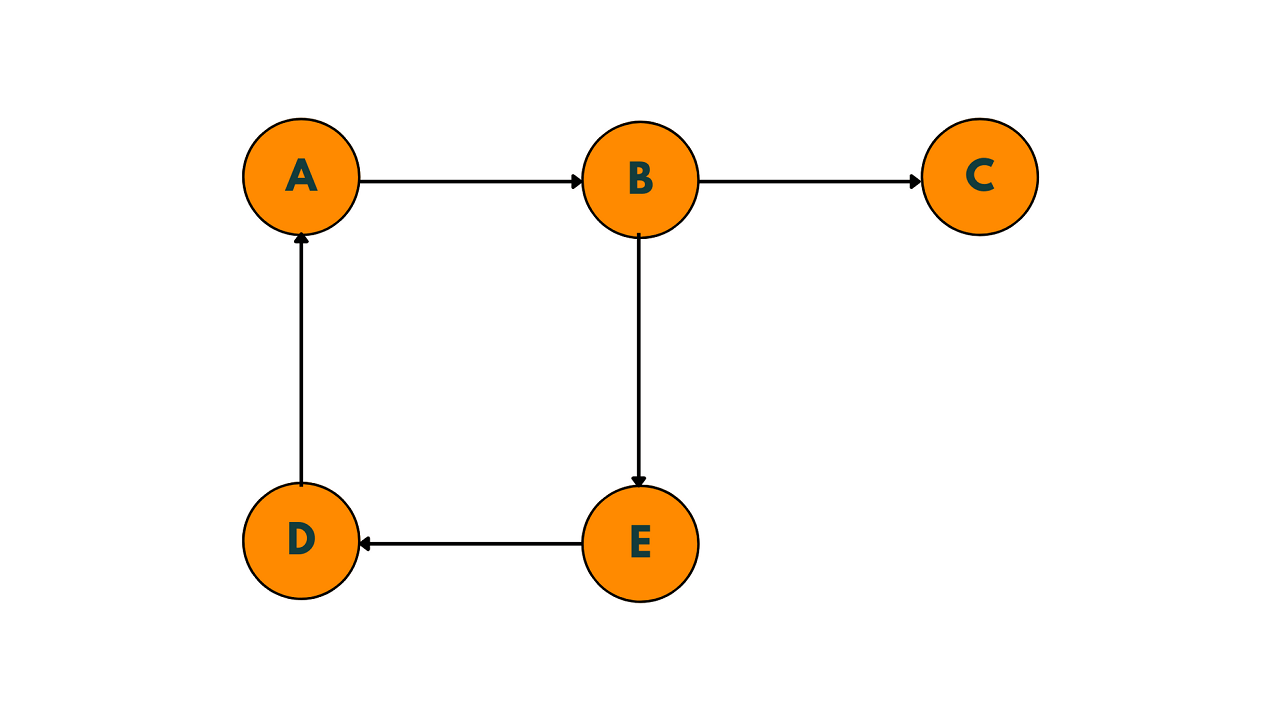# Detect Cycle in a Directed Graph using DFS & BFS (with code)

In this article, we will discuss how to detect a cycle in a directed graph. A directed graph is a collection of nodes and edges where the edges have a direction. Detecting a cycle in a directed graph is a common problem in computer science.

## How to Detect Cycle in a Directed Graph?

A cycle is a path in a graph where the starting and ending vertices are the same. In a directed graph, a cycle is a path where the direction of the edges is such that it forms a closed loop. In other words, a cycle is a path where the edges form a closed loop, and no vertex is visited more than once.

Detecting a cycle in a directed graph can be done using different algorithms. One of the most common algorithms is the Depth First Search (DFS) algorithm. Let's understand the DFS algorithm.

### Detect Cycle in a Directed Graph using DFS

First, let's revise what is DFS. Depth First Search is a graph traversal algorithm that visits all the vertices (nodes) of a graph by exploring as far as possible along each branch before backtracking. It starts at a given node and explores as far as possible along each branch before backtracking to explore the next branch.DFS can be implemented using recursion or a stack data structure. The recursive implementation is simpler, but may not be as efficient for very large graphs.

One of the advantages of DFS is that it uses less memory than BFS because it only needs to keep track of a single path in the graph at a time. However, it may not find the shortest path between two nodes

One of the most common algorithms for detecting cycles in directed graphs is the Depth First Search (DFS) algorithm. The algorithm works by traversing the graph in a depth-first manner, starting from a given node, and visiting all the nodes in the graph. During the traversal, the DFS algorithm keeps track of the visited nodes and the nodes that have been visited but have not yet been fully explored.

Here are the steps for the DFS algorithm to detect a cycle in a directed graph:

1. Initialize all nodes as unvisited (i.e., white).
2. Pick an unvisited node and mark it as currently being explored (i.e., gray).
3. For each adjacent node of the current node: a. If the adjacent node is white, mark it as currently being explored (i.e., gray) and recursively visit it. b. If the adjacent node is gray, then a cycle has been detected. c. If the adjacent node is black, then it has already been fully explored, so move on to the next adjacent node.
4. Once all adjacent nodes have been visited, mark the current node as fully explored (i.e., black).
5. Repeat steps 2-4 for all unvisited nodes in the graph.

During the DFS traversal, if we encounter a node that is currently being explored (i.e., has the color gray), then we have found a cycle in the graph. This is because we have found a path that starts and ends at the same node and includes a back edge. A back edge is an edge that connects a node to a previously explored node that is not its parent in the DFS tree.

The time complexity of the DFS algorithm for detecting cycles in a directed graph is O(V+E), where V is the number of vertices and E is the number of edges in the graph.

Here's an implementation of the DFS algorithm in C++ to detect cycles in a directed graph:

```#include<iostream>
#include<vector>
#include<unordered_map>

using namespace std;

enum class Color {
WHITE,
GRAY,
BLACK
};

bool hasCycleDFS(const unordered_map<int, vector<int>>& graph, unordered_map<int, Color>& visited, int node) {
visited[node] = Color::GRAY;

for (int neighbor : graph.at(node)) {
if (visited[neighbor] == Color::GRAY) {
return true;
}
else if (visited[neighbor] == Color::WHITE) {
if (hasCycleDFS(graph, visited, neighbor)) {
return true;
}
}
}

visited[node] = Color::BLACK;
return false;
}

bool hasCycle(const unordered_map<int, vector<int>>& graph) {
unordered_map<int, Color> visited;

for (const auto& entry : graph) {
visited[entry.first] = Color::WHITE;
}

for (const auto& entry : graph) {
if (visited[entry.first] == Color::WHITE) {
if (hasCycleDFS(graph, visited, entry.first)) {
return true;
}
}
}

return false;
}

int main() {
// Example usage
unordered_map<int, vector<int>> graph {
{0, {1}},
{1, {2}},
{2, {3}},
{3, {1}}
};

if (hasCycle(graph)) {
cout << "The graph has a cycle" << endl;
}
else {
cout << "The graph does not have a cycle" << endl;
}

return 0;
}
```

Output:

```The graph has a cycle
```

The input `graph` is an unordered map that represents the directed graph, where the keys are the nodes, and the values are vectors of the nodes that are adjacent to the key node.

The `hasCycleDFS` the function performs a DFS traversal starting from the given `node`. The `visited` the unordered map keeps track of the status of each node in the DFS traversal, where a node can have one of three statuses: `Color::WHITE` if it has not been visited yet, `Color::GRAY` if it is currently being explored, and `Color::BLACK` if it has been fully explored.

For each adjacent `neighbor` of the `node`, the function checks if it has already been visited (`visited[neighbor] == Color::GRAY`) and returns `true` if a cycle is detected. Otherwise, if the `neighbor` has not been visited yet (`visited[neighbor] == Color::WHITE`), the function recursively calls itself on the `neighbor`.

After all adjacent nodes have been explored, the `node` is marked as fully explored (`visited[node] = Color::BLACK`), and the function returns `false`.

The `hasCycle` the function iterates through all nodes in the graph and calls `hasCycleDFS` on each unvisited node. If a cycle is detected during any of the DFS traversals, the function immediately returns `true`. Otherwise, if all DFS traversals have been completed without finding a cycle, the function returns `false`.

In the `main` function, we provide an example usage of the `hasCycle` function on a sample-directed graph. If the graph has a cycle, the function outputs "The graph has a cycle". Otherwise, if the graph does not have a cycle, the function outputs "The graph does not have a cycle".

Here's a similar code in Python:

```from collections import defaultdict

# Function to perform DFS traversal
def DFS(graph, node, visited, rec_stack):
# Mark the current node as visited and add it to the recursion stack
visited[node] = True
rec_stack[node] = True

# Recur for all the neighboring vertices
for neighbor in graph[node]:
# If neighbor is not visited, then recur for it
if not visited[neighbor]:
if DFS(graph, neighbor, visited, rec_stack):
return True
# If neighbor is already visited and present in recursion stack, then cycle exists
elif rec_stack[neighbor]:
return True

# Remove the node from recursion stack
rec_stack[node] = False
return False

# Function to detect cycle in directed graph using DFS
def detect_cycle_DFS(graph):
# Create a visited set and a recursion stack
visited = {node: False for node in graph}
rec_stack = {node: False for node in graph}

# Perform DFS traversal for all nodes to detect cycle
for node in graph:
if not visited[node]:
if DFS(graph, node, visited, rec_stack):
return True

return False

# Example directed graph
graph = {
'A': ['B'],
'B': ['C', 'E'],
'C': [],
'D': ['A', 'E'],
'E': ['D']
}

# Detect cycle in graph using DFS
if detect_cycle_DFS(graph):
print("Cycle exists in graph")
else:
print("Cycle does not exist in graph")
```

Output:

```Cycle exists in graph
```

The above code takes a graph as input in the form of a dictionary of adjacency lists, where the keys are the nodes and the values are lists of their neighboring nodes. It then performs DFS traversal starting from each node to detect if there exists a cycle in the graph. If a cycle is found, the function returns True; otherwise, it returns False.

### Detect Cycle in Directed Graph using BFS

Detecting cycles in directed graphs can also be done using the Breadth First Search (BFS) algorithm. In this approach, we perform a BFS traversal of the graph, and if at any point we encounter a node that has already been visited and is present in the BFS queue, we can conclude that there exists a cycle in the graph.

Here is an example of how to detect cycles in directed graphs using BFS. Consider the following directed graph:To detect cycles in this graph using BFS, we start by initializing an empty queue and a visited set. We then enqueue the starting node and mark it as visited. We then repeatedly dequeue nodes from the queue and add all their unvisited neighbors to the queue, marking them as visited.

While doing this, we keep track of the parent node for each node we visit, so that we can trace back the path to any cycle we find.

Starting at node A, we enqueue it and mark it as visited. We then dequeue node A and add its neighbors B and D to the queue. We mark both B and D as visited and set their parent nodes as A. We continue dequeuing and enqueuing nodes until the queue becomes empty.

Now, let's suppose we start the BFS traversal again, but this time starting at node E instead of A. We enqueue node E and mark it as visited. We then dequeue node E and add its neighbors B and D to the queue. However, B is already in the visited set, and its parent node is not E, but A. Therefore, we know that there exists a cycle in the graph, which is A -> B -> E -> D -> A.

Thus, by performing BFS on the directed graph, we were able to detect the cycle in the graph.

Also, learn how to detect cycle in an undirected graph here.

## Conclusion

Overall, the problem of detecting cycles in directed graphs has numerous applications in various fields, including Network Analysis, Identifying the flow of current in a circuit, and Biological Systems. In finance, detecting cycles in directed graphs is useful in analyzing financial networks, including stock market networks, bank networks, and credit networks.

### FavTutor - 24x7 Live Coding Help from Expert Tutors!